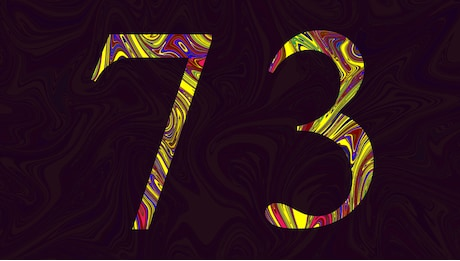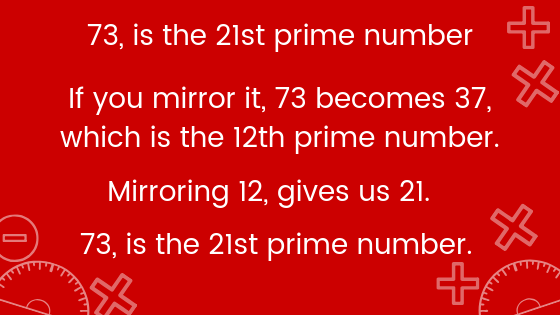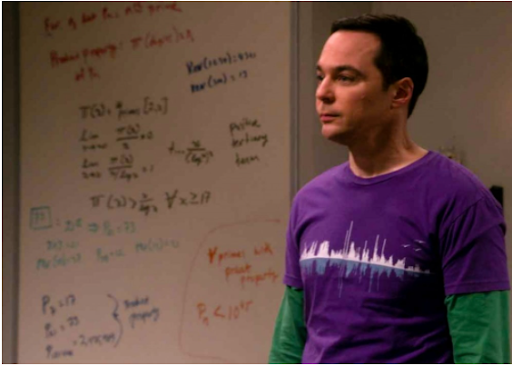Math Puzzles
What makes this number so special?
27.4k views

 1 Introduction 2 Properties of Prime Numbers? 3 Which is the smallest prime number? 4 Which is the largest prime number? 5 Is 73 a prime number? 6 Why 73 is a special number? 7 How this special number 73 was discovered? 8 Conclusion 9 About Cuemath 10 FAQ’s 11 External References

##12th November 2020

## Introduction

### What are prime numbers?

A prime number is a natural number greater than 1 that is not a product of two smaller natural numbers. A natural number greater than 1 that is not prime is called a composite number.

For Example:

2, 3, 5, 7 and 11 are the first few prime numbers.

### History of prime number?

In about 200 BC the Greek Eratosthenes devised an algorithm for calculating primes called the Sieve of Eratosthenes.

There is then a long gap in the history of prime numbers during what is usually called the Dark Ages.

The next important developments were made by Fermat at the beginning of the 17th Century.

### list of prime numbers from 1 to 100?

2, 3, 5, 7, 11, 13, 17, 19, 23, 29, 31, 37, 41, 43, 47, 53, 59, 61, 67, 71, 73, 79, 83, 89, 97

### What makes this number so special-PDF

What makes this number so special? 73 and why is it so special. It actually holds a lot of mind-boggling facts. Here is a downloadable PDF to explore more.

## Properties of Prime Numbers?

Some of the properties of prime numbers are:

• Every number greater than 1 can be divided by at least one prime number.
• Every even positive integer greater than 2 can be expressed as the sum of two primes.
• Except 2, all other prime numbers are odd. In other words, we can say that 2 is the only even prime number.
• Two prime numbers are always coprime to each other.

Each composite number can be factored into prime factors and individually all of these are unique in nature.

## Which is the smallest prime number?

The smallest prime number defined by modern mathematicians is 2. To be prime, a number must be divisible only by 1 and the number itself which is fulfilled by the number 2.

## Which is the largest prime number?

As of January 2020, the largest known prime number is 2^(82,589,933) – 1 a number which has 24,862,048 digits. It was found by the Great Internet Mersenne Prime Search (GIMPS) in 2018.

## Is 73 a prime number?

It is possible to find out using mathematical methods whether a given integer is a prime number or not.

For 73, the answer is: yes, 73 is a prime number because it has only two distinct divisors: 1 and itself (73).

As a consequence, 73 is only a multiple of 1 and 73.

Since 73 is a prime number, 73 is also a deficient number, that is to say 73 is a natural integer that is strictly larger than the sum of its proper divisors, i.e., the divisors of 73 without 73 itself (that is 1, by definition!).

### Parity of 73

73 is an odd number because it is not evenly divisible by 2.

## Why 73 is a special number?## How this special number 73 was discovered?A proof co-authored by Dartmouth mathematics professor emeritus Carl Pomerance and Morningside College mathematics professor Chris Spicer appeared on an episode of the television series, “The Big Bang Theory” on April 18. The proof, which was featured on a whiteboard in the background of the show, reveals the uniqueness of the number 73.

Sheldon Cooper, one of the main characters on “The Big Bang Theory,” first expressed his affinity for the number 73 during the show’s 73rd episode, “The Alien Parasite Hypothesis,” which aired in 2010.

“The best number is 73,” Cooper explained in the episode. “Why? 73 is the 21st prime number. Its mirror, 37, is the 12th, and its mirror, 21, is the product of multiplying seven and three ... and in binary, 73 is a palindrome, 1001001, which backwards is 1001001.”

After the episode aired, Spicer, along with two Morningside College students, Jessie Byrnes and Alyssa Turnquist, published a 2015 article in Math Horizons, an undergraduate-focused journal, discussing the potential of creating a proof for Cooper’s claims.

“I really was just watching an episode of ‘The Big Bang Theory’ back in 2014 where Sheldon made his claim,” Spicer said. “I was intrigued, so I asked a couple students of mine to see if they could prove it.”

Pomerance said that he first discovered the Math Horizons article back in 2015 and sent a brief email to Spicer. However, he did not follow-up with Spicer until last summer, when he contacted Spicer about working together to create a proof. Spicer said that after about 130 emails exchanged between the two mathematicians, they had figured out the proof.

## Conclusion

Been amazed by knowing the logic behind the special number 73 math is magical in many ways. They hold great mathematical mysteries in them and have continuously piqued the interests of mathematicians all over the world ever since mathematician Elucid of Alexandria quoted that: ‘Prime numbers have no end.’

What type of a number is 73?

73 (seventy-three) is the natural number following 72 and preceding 74. In English, it is the smallest natural number with twelve letters in its spelled out name.

You can know more about prime numbers.

What are prime numbers, and why are they so vital to modern life?

Prime numbers are cool. As Carl Sagan points out so eloquently in the novel Contact, there’s a certain importance to their status as the most fundamental building block of all numbers, which are themselves the building blocks of our understanding of the universe.

Cuemath, a student-friendly mathematics platform, conducts regular Online Classes for academics and skill-development and their Mental Math App, on both iOS and Android, is a one-stop solution for kids to develop multiple skills.Understand the Cuemath fee structure and sign up for a free trial.

## What is the prime factorization of 73?

Prime factorization: 73 is prime. The exponent of prime number 73 is 1. Adding 1 to that
exponent we get (1 + 1) = 2. Therefore 73 has exactly 2 factors

## What numbers can 73 be divided by?

73 is a prime number from 1-100. 73 has 2 factors, 1 and 73.

## Why are they called "prime" numbers?

They're "prime" in the sense that they "come first", in that we can get all of the others (composite numbers) by combining them through multiplication. When Euclid first wrote about them in his Elements, he used the Greek word "πρωτος" ("protos"), early translations of which were given as "prime", the word that has been used ever since.

Related Articles